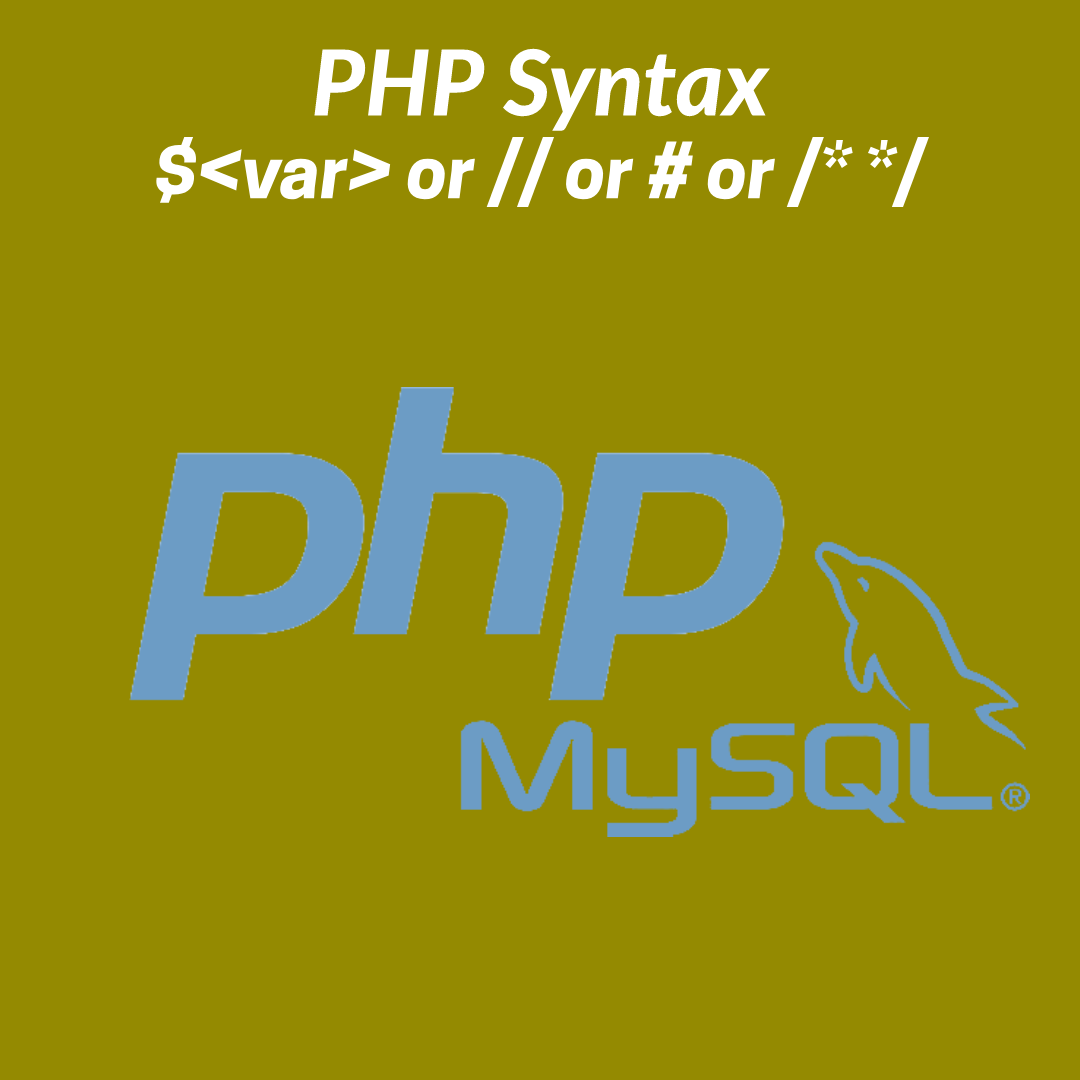# What are the basic syntax of PHP?To make a project using PHP first need to know the basic syntax of PHP. that is how to make a comment a line or multiple lines, or how to print an array or a string and also how to concatenate multiple strings.

### How to make a single line or multiple lines commenting?

Using PHP we can make a single line comment by putting # or // before the line. and to make multiple line commenting have to put the line between /* and */ the example is given below

``````# single line commenting
// also a single line commenting
/* its a process to make
multiple line commenting
that is the line between
slash star and star slash
will be specify as commented. */``````

### How to print a string?

In PHP a string can be print by using echo keyword and in this case, any latter can be upper or lower it will not make any effect that is echo is not case sensitive. An example is given below.

``````<?php
EcHo "Print the value";//Print the value
ECHO "Print the value";//Print the value
echo "Print the value";//Print the value
?>``````

if the portion to print is inside a single quotation then it will print exactly what is inside the single quote, maybe its a variable also. But if it’s inside double quote then it will print the value assigned to the variable, the example is as below.

``````<?php
\$i = 10;
echo "The value of i = \$i"; //The value of i = 10
echo 'The value of i = \$i'; //The value of i = \$i
?>``````

Also using the print function user can be able to print a string and the variable inside it according to the double quote and single quote method as above. That is a single quote that will print an exact string and a double quote will print the value of variable also. an example is given below

``````<?php
print("It will print the line inside the brasses and quote if it is not a variable"); //It will print the line inside the brasses and quote if it is not a variable
?>``````

### How to print an array or object?

to print an array we can use ver_dump or print_r function both will print the array with its key but ver_dump will print the details information about the array or object like its array length, the string length of the value, etc. below is the example of ver_dump and print_r function.

``````<?php
\$arrayName = array(
'a' => 'a1',
'b' => 'ab1',
'c' => 'av1',
);

print_r(\$arrayName);

ver_dump(\$arrayName);
?>``````

### How to concatenate two or more string.

Using PHP we can concatenate two or more string or veritable or value by putting a dot ( . ) between them. an example is given below on how to concatenate two or more string.

``````<?php
\$i = 10;
echo 'The value of i = ' . \$i . ' and it will print the value';
//The value of i = 10 and it will print the value
?>``````

I hope that the post has helped you to learn about the PHP basic syntax. and you have learned how to make comment or print or concatenate.